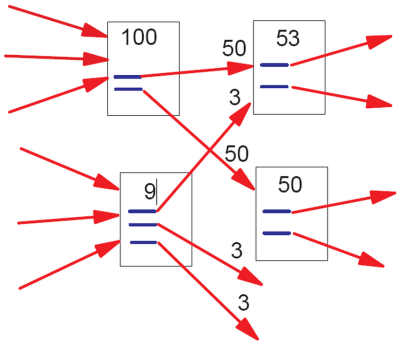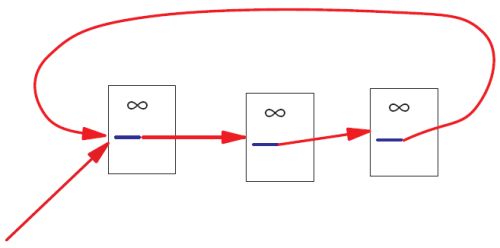This site contains: mathematics courses and book; covers: image analysis, data analysis, and discrete modelling; provides: image analysis software. Created and run by Peter Saveliev.

# PageRank

Why it's interesting?

My interest in data analysis has lead me to read about its uses in Internet search, then to Google search algorithm, then finally to its core, the PageRank.

PageRank is a way to compute the importance of web-pages based on their incoming and outgoing links.

PageRank is a mathematical idea that "made" Google's web search algorithm. In fact, PageRank was the search algorithm, initially. Even with so many things added, PageRank remains the cornerstone of web search. Therefore, its problems become problems of web search and, hence, our problems. These problems, I believe, are mathematical in nature.

Google: "PageRank thinks of links as 'votes', where a page linking to another page is essentially casting a vote for that page... PageRank also considers the importance of each page that casts a vote, as votes from some pages are considered to have greater value, thus giving the linked page greater value."

Wikipedia: "PageRank is a probability distribution used to represent the likelihood that a person randomly clicking on links will arrive at any particular page. ... It is assumed in several research papers that the distribution is evenly divided among all documents in the collection at the beginning of the computational process. The PageRank computations require several passes, called "iterations", through the collection to adjust approximate PageRank values to more closely reflect the theoretical true value."

As a mathematical idea I find PageRank very unsatisfying. The reason is that PageRank, as well its derivatives, uses made-up parameters.

Let's recall the basics of PageRank.

According to Google's own Matt Cutts (the pictures come from the original paper):"In the image above, the lower-left document has “nine points of PageRank” and three outgoing links. The resulting PageRank flow along each outgoing link is consequently nine divided by three = three points of PageRank.

That simplistic model doesn't work perfectly, however. Imagine if there were a loop:No PageRank would ever escape from the loop, and as incoming PageRank continued to flow into the loop, eventually the PageRank in that loop would reach infinity. Infinite PageRank isn’t that helpful [smiley face] so Larry and Sergey introduced a decay factor–you could think of it as 10-15% of the PageRank on any given page disappearing before the PageRank flows along the outlinks. In the random surfer model, that decay factor is as if the random surfer got bored and decided to head for a completely different page."

Seems like a reasonable model?

At a glance, yes.

The first sign of trouble is how the "decay factor" is introduced. The trick made me laugh. I am imagining the two Google guys thinking: "OK, we are getting $$1+1+1+...$$ But it goes to infinity! What to do, what to do? Eureka! Let's make the sequence into $$1+r+r^2+r^3+...,$$ i.e., a geometric progression!"

Now, what should $r$ be? Of course, they remember calc2 and know that the "ratio" $r$ should be less than $1$ if you want the series to converge.

"Let's pick $r=.85$! Why? What do you mean why? This is the most natural choice!"

This number is a made-up parameter in the sense that there is no justification for choosing it over another value.

Let's be clear, if you create new mathematics, you should expect it to be subject to mathematical scrutiny.

A few topics are presented: